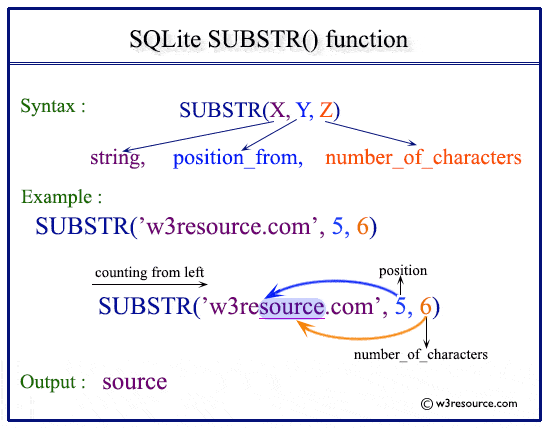# SQLite substr() function

## Description

SQLite substr() returns the specified number of characters from a particular position of a given string.

Syntax:

```substr(X,Y,Z);
substr(X,Y);
```

Arguments:

Name Description
X A string from which a substring is to be returned.
Y An integer indicating a string position within the string X.
Z An integer indicating a number of characters to be returned.

If Z is omitted then substr(X,Y) returns all characters through the end of the string X beginning with the Y-th. The left-most character of X is number 1. If Y is negative then the first character of the substring is found by counting from the right rather than the left. If Z is negative then the abs(Z) characters preceding the Y-th character are returned.

SQLite Version: 3.8.5

Pictorial PresentationExample-1:SQLite substr() function

The following SQLite statement returns 3 numbers of characters from the 4th position of the string ‘w3resource’.

``````SELECT substr('w3resource',4,3);
```
```

Here is the output.

Sample Output:

```SUBSTR('w3resource',4,3)
------------------------
eso
```

Example-2: SQLite substr() using table

Sample table: employees

The following SQLite statement returns 4 numbers of characters from the 4th position of the column first_name for those employees which belongs to the department 60 from the table employees.

``````SELECT first_name,substr(first_name,3,4)
FROM employees
WHERE department_id=60;
```
```

Here is the output.

Sample Output:

```first_name  substr(first_name,3,4)
----------  ----------------------
Alexander   exan
Bruce       uce
David       vid
Valli       lli
Diana       ana
```

Example-3: SQLite substr() function extracts rest characters from a specific position

Sample table: employees

The following SQLite statement returns the rest of the characters from the 3rd position of the column first_name for those employees who belongs to the department 100 from the table employees.

``````SELECT first_name, substr(first_name,3)
FROM employees
WHERE department_id=100;
```
```

Here is the result.

Sample Output:

```first_name  substr(first_name,3)
----------  --------------------
Nancy       ncy
Daniel      niel
John        hn
Ismael      mael
Jose Manue  se Manuel
Luis        is
```

Example-4:SQLite substr() extracts from negative position

Sample table: employees

The following SQLite statement returns the rest of the characters from the 3rd position from the end (since -3 is used) of the column first_name for those employees who belong to the department 100 from the table employees.

``````SELECT first_name, SUBSTR(first_name ,-3)
FROM employees
WHERE department_id=100;
```
```

Here is the result.

Sample Output:

```first_name  SUBSTR(first_name ,-3)
----------  ----------------------
Nancy       ncy
Daniel      iel
John        ohn
Ismael      ael
Jose Manue  uel
Luis        uis
```

Example-5: SQLite substr() extracting from the end

Sample table: employees

The following SQLite statement returns 3 characters from the 15th position from the end (since -15 is used) of the column first_name for those employees who belongs to the department 100 from the table employees.

``````SELECT first_name, SUBSTR(first_name ,-6,3)
FROM employees
WHERE department_id=100;
```
```

Here is the result.

Sample Output:

```first_name  SUBSTR(first_name ,-6,3)
----------  ------------------------
Nancy       Na
Daniel      Dan
John        J
Ismael      Ism
Jose Manue  Man
Luis        L
```

Example-6 :SQLite substr() function

The following SQLite statement returns 3 characters from the string ‘w3resource’. Here the starting position is a positive number but the value for retrieving character is negative, so the abs(-3) characters preceding the 4th character are returned.

``````SELECT substr('w3resource',4,-3);
```
```

Here is the output.

Sample Output:

```substr('w3resource',4,-3)
-------------------------
w3r
```

Previous: rtrim()
Next: trim()

﻿